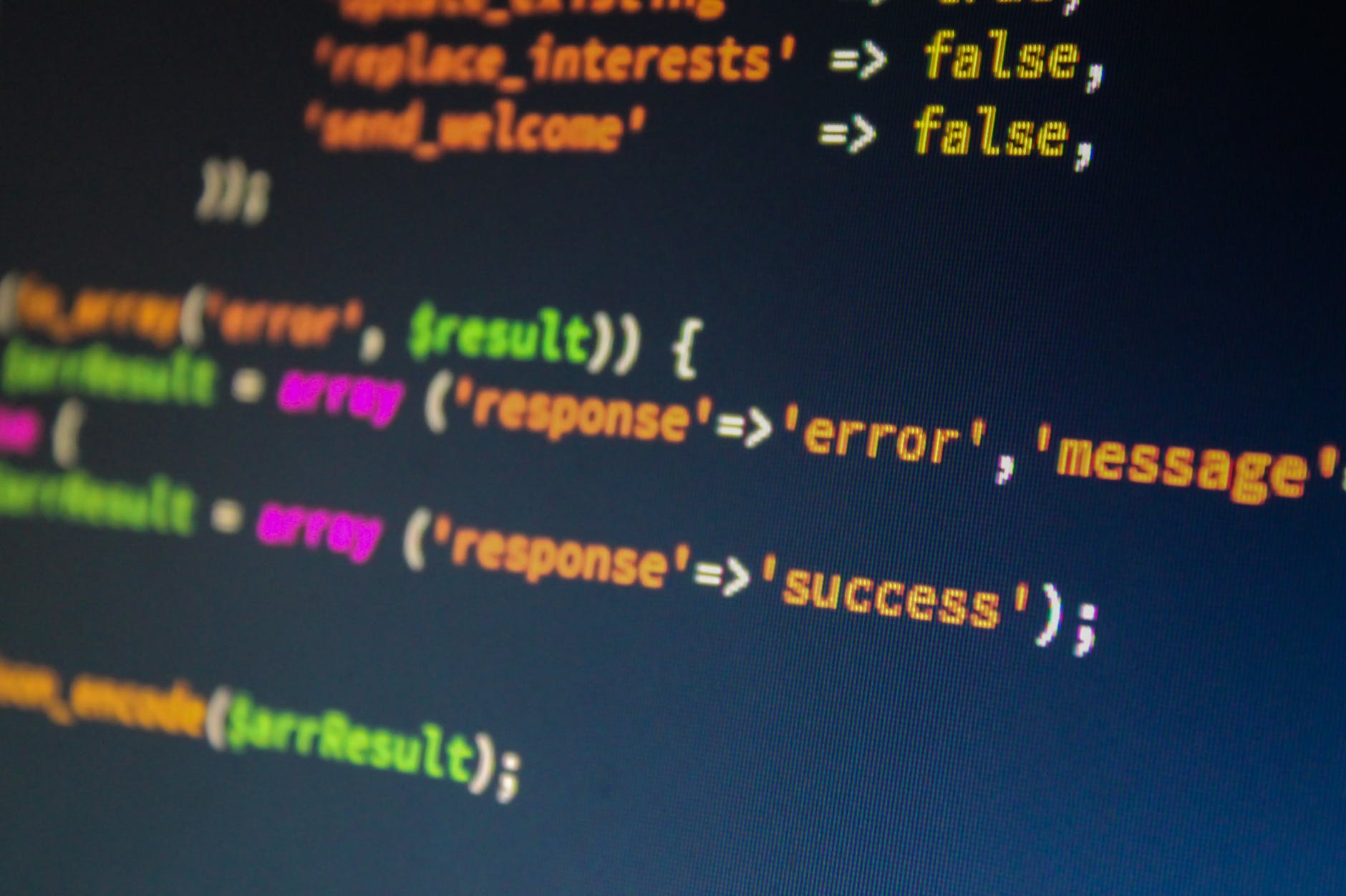# Making a Function in Excel VIDEO

Functions allow the user to automate redundant tasks that need to be completed. When you first begin making them sometimes it seems as those making the function takes more time than doing it manually. However, everything gets easier with practice. In the video below we will learn how to develop functions in Excel.# Making Functions in Python

Efficiency is important when writing code. Why would you type something a second time if you do not have to? One way coders save time, reduce error, and make their code easier to read is through the use of functions.Functions are a way of storing code so that it can be reused without having to retype or code it again. By saving a piece of reusable code as a function you only have to call the function in order to use it again. This improves efficiency and reliability of your code. Functions simply take an input, rearrange the input however it is supposed to, and provide an output. In this post, we will look at how to make a function using Python.

Simple Function

A function requires the minimum information

• A name
• Determines if any requirements (arguments) are needed
• The actual action the function is supposed to do to the input

We will now make a simple function as shown in the code below.

```def example():
print("My first function")```

In the code above we have the three pieces of information.

• The name of the function is “example” and you set the name using the “def” command.
• There are no requirements or arguments in this function. This is why the parentheses are empty. This will make more sense later.
• What this function does is use the “print” function to print the string “My first function”

We will now use the function by calling it in the example below.

```example()
My first function```

As you can see, when we call the function it simply prints the string. This function is not that impressive but it shows you how functions work.

Functions with Arguments

Arguments are found with the parentheses of a function. They are placeholders for information that you must supply in order for the function to work. Below is an example.

```def example(info):
print(info)```

Now our “example” function has a required argument called “info” we must always put something in place of this for the function to run. Below is an example of us calling the “example” function with a string in place of the argument  “info”.

```example("My second function")
My second function```

You can see that the function simply printed what we placed in the paratheses. If we had left the parentheses empty we would have gotten an error message. You can try that yourself.

You can assign a default value to your argument. This is useful if people do not provide their own value. Below we create the same function but with a default value for the argument.

```def example(info="You forgot to give a value"):
print(info)```

We will now call it but we will not include the argument

```example()
You forgot to give a value```

return and print

When creating functions, it is common to have to decide when to use the “return” or “print” function. Below are some guidelines

• Print is for people. If a person only needs to see the output without any other execution the print is a good choice.
• Return is appropriate when sending the data back to the caller for additional execution. For example, using one function before using a second function

If you take any of the examples and use “return” instead of “print” they will still work so the difference between “return” and “print” depends on the ultimate purpose of the application.

Conclusion

Functions play a critical role in making useful applications. This is due to their ability to save time for coders. THere are several concepts to keep in mind when developing functions. Understanding these ideas is important for future success.